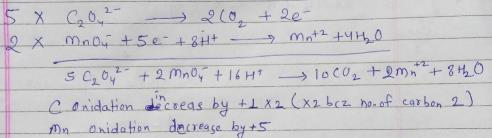Courses

# Test: Balancing Redox Reactions

## 5 Questions MCQ Test Chemistry Class 11 | Test: Balancing Redox Reactions

Description
This mock test of Test: Balancing Redox Reactions for JEE helps you for every JEE entrance exam. This contains 5 Multiple Choice Questions for JEE Test: Balancing Redox Reactions (mcq) to study with solutions a complete question bank. The solved questions answers in this Test: Balancing Redox Reactions quiz give you a good mix of easy questions and tough questions. JEE students definitely take this Test: Balancing Redox Reactions exercise for a better result in the exam. You can find other Test: Balancing Redox Reactions extra questions, long questions & short questions for JEE on EduRev as well by searching above.
QUESTION: 1

Solution:
QUESTION: 2

### The oxidation half reaction for following reaction is Fe2+(aq) + Cr2O72-(aq) → Fe3+ (aq) + Cr3+(aq)

Solution:

Oxidation half reaction for a reaction is that reaction which gives us the reactant and product formed after the oxidation of the reactant. In this case Fe+2 oxidizes itself to Fe+3 and so the oxidation of Fe+2 is oxidation half reaction. Option d correct.

QUESTION: 3

### In this method, the two half equations are balanced separately and then added together to give balanced equation

Solution:

In the ion-electron method (also called the half-reaction method), the redox equation is separated into two half-equations - one for oxidation and one for reduction. Each of these half-reactions is balanced separately and then combined to give the balanced redox equation.

QUESTION: 4

The process in which the strength of an unknown solution is calculated using a known standard solution.

Solution:

A titration is a technique used to work out the concentration of an unknown solution based on its chemical reaction with a solution of known concentration. The process usually involves adding the known solution (the titrant) to a known quantity of the unknown solution (the analyte) until the reaction is complete.

QUESTION: 5

For the redox reaction

MnO4 + C2O42- + H+ → Mn2+ + CO2 + H2O
The correct coefficients of the reactants for the balanced reaction are:

Solution: